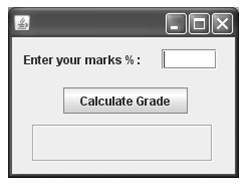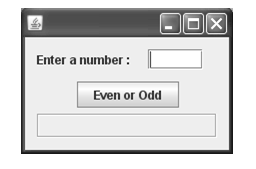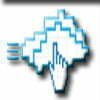Home » Sample Papers » Sample Paper Economics 11 » Sample Paper of Information Practice 2014 for class 11, CBSE. Paper No.2

# Sample Paper of Information Practice 2014 for class 11, CBSE. Paper No.2

Sample paper – 2014
Class – XI
Subject –
Informatics Practices

Max. Marks : 70                                                                                   Time            : 3 Hrs.

Note :  Answer all the questions.

SECTION – A ( 20 Marks )
Q.1 Answer the following questions :                                                                                         ( 5 x 2 = 10 )
(a) What are the Strengths and Weakness of a Computer.
(b) What are Input Devices ? Explain any three.
(c) Explain the working of a dot-matrix printer.
(d) Explain the various types of  Operating Systems.
(e) Name some port types briefly.
Q.2 What are the differences between :                                                                                        ( 5 x 1 = 5 )
(a) Magnetic disks and Optical disks      (b)  Data and Information
(c) Package and Utilities                         (d)  Firmware and Malware      (e)  RAM and ROM
Q.3 Define the terms :                                                                                                                   ( 3 x 1 = 3 )
Q.4 Write the full forms of the following terms :                                                                     ( 4 x 1/2  = 2 )
(a) OMR           (b)  LCD        (c)  VDU          (d)   CD – ROM
SECTION B ( 10 Marks )
Q.5 Answer the following questions :
(a) What are Identifiers ?  What are the Identifiers forming rules in Java ? 2
(b) List out all the Operators in Java ? Explain briefly. 2
(c) Write a note on Java Data Types ? 2
(d) What is Type Casting ? Explain with an example. 1
(e) What will be the result of a = 5 / 3, if a is    (i) float     (ii) int  ? 1
(f) What will be the value of expression j = – – k + 2 * k . if k is 20 initially ? 1
(g) The user wants to store the values, which data type should the user take for representing.(i)  23.197           (ii)  ‘a’ 1
1SECTION C  ( 30 Marks )
Q.6 Answer the following questions :
(a) Find the output of the following code fragments?
(i)          int s = 14 ;if (s < 20 )

System . out . print ( “Under“ ) ;

else

System . out . print ( “Over“ ) ;

System . out . println ( “the limit“ ) ;

2
(ii)        int i = 3 , n = 0 ;while ( i < 4 )

{

n + + ;

i – – ;

}

System . out . println (n ) ;

2
(b) Find out the error in the following code fragment and rewrite the corrected code with explanation.while ( i < j ) ;   {

System . out . println ( i * j ) ;

i + + ;

}

2
(c) How many times the following loop is executed ? Explain.int x = 5 , y = 50 ;

while ( x < = y )

{

x = y / x ;

}

2
(d) Write equivalent Java expression for the following expressionÖ a2 + b2 + c2 1
(e) Compare if and switch statements. 1
(a) Design a GUI, with a TextField , Label and a Button. Write the code for the Button to accept a integer into the TextField from the user and then calculate and display the factorial of a given number in the Label provided. Using do-while loop. 2
2
(b) Rewrite the following fragment using while loop :int sum = 0 ;

for ( int i  = 0 ; i < = 5 ; + + i )

sum = sum + i ;

System . out . println ( “Sum is : “ + sum ) ;

2
(c) Rewrite the following fragment using switch :if ( ch = = ‘E’ )

eastern + + ;

if ( ch = = ‘W’ )

western + + ;

if ( ch = = ‘N’ )

northern + + ;

if ( ch = = ‘S’ )

southern + + ;

else

unknown + + ;

2
(d) Design a GUI, with a TextField , Label and a Button. Write the code for the Button to accept a integer number into the TextField from the user and then check wither the given number is palindrome or not and display the same as “Palindrome” or “Not a Palindrome” in the Label provided. Using while loop and if-else statement. 2
(e) Design a GUI, with a TextField , TextArea and a Button. Write the code for the Button to accept a integer number into the TextField from the user and then display the multiplication table of the given number in the TextArea provided. Using for loop. 2
(a) For the following GUI application. The percentage marks are to be entered in the TextField and upon clicking the Button, corresponding grade ( as per following rules ) should be displayed in the Label.

 Marks Percentage Grade > = 90 A + + 80 – 90 A + 75 – 80 A 60 – 75 B 50 – 60 C 40 – 50 D < 40 Fail4
3
(b) Create a GUI application that allows the user to enter some text in a Textfield. The application has three Buttons : Move Text , Clear and Exit.When the user clicks at(i)   Move Text Button, the text should moved to the OutputLabel. And upon clicking 2
(ii)  Clear Button, the text in the Textfield as well as in the OutputLabel should be cleared. 1
(iii)  Exit Button, the application should end. 1
(c) Using the following  GUI, Write the code for the Button check wither the given number is “Even” or “Odd” and display the same in the Label provided. Using if-else.2
SECTION D ( 10 Marks )
Q9. Read the following case study and answer the questions that follows :
The FOR U SHOP has computerized its billing. A new bill is generated for each customer. The shop allows three different payment modes. The discount is given based on the payment mode. The following is the data entry screen used at to generate the bill.4
The list of controls for the above form is as follows :

 Object Type Object Name Description Frame FrmDiscount The main frame Text Field TxtAmt To input the total Shopping Amount TxtDisc To display Discount Amount TxtNet To display Net Price Radio Button OptCash To select mode of payment as cash OptCheque To select mode of payment as cheque OptCredit To select mode of payment as credit card Ok Button CmdCalc To calculate Discount and Net Price CmdClear To clear all Text Fields CmdExit To exit from the application
(a) Write the code for the CmdClear Button to clear all the Text Fields 1
(b) Write the code for  formWindowActivated event of FrmDiscount so as to
(i)  disable the TxtDisc and the TxtNet Text Fields 1
(ii) set default choice in the Radio Button as Cash 1
(iii) set the Focus to the TxtAmt  Text Fields 1
(c) Write the code for the TxtAmtFocusLost event of the TxtAmt Text Field to ensure that the user does not enter a negative or a zero value. If a negative or zero value is entered then the Text Field should be made blank and a warning message should be displayed. 2
(d) Write the code for the CmdCalc Button to display the Discount Amount and Net Price in the TxtDisc and the TxtNet Text Fields respectively. Note that Net Price is calculated as Shopping Amount – Discount Amount and the discount is calculated based on the credit card and the total shopping amount according to the following table.

 Credit Card Type Shopping Amount Discount Cash < 10000 20 % >= 10000 25 % Cheque < 15000 10 % >= 15000 15 % Credit Card < 10000 10 % >= 10000 12 %
3
(e) Write the code for CmdExit Ok button to display a message “Thank You “ and exit out of the application. 1
5

Paper Submitted by

Name: Lakshmi Pulikollu

Email: lakshmi.ip@rediffmail.com

Phone No.      9492921745The main objective of this website is to provide quality study material to all students (from 1st to 12th class of any board) irrespective of their background as our motto is “Education for Everyone”. It is also a very good platform for teachers who want to share their valuable knowledge.